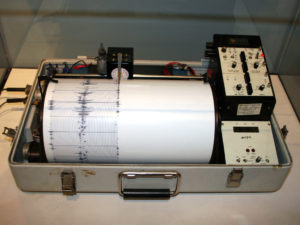## Why Learn About Exponential and Logarithmic Equations?

Joan enjoyed talking to her grandfather so much, that the next Sunday, she made sure she she would be there when he came to dinner. This time, though, she prepared a question for her grandfather. She had been learning about logarithmic and exponential functions in her math class and wanted to see if she could stump her grandfather with a math question.A Kinemetrics Seismograph formerly used by the United States Department of the Interior.

One of the questions in Joan’s homework on exponential and logarithmic functions had been about how to calculate the Richter scale measure of the magnitude of an earthquake. The following formula was given in her book:

$R=\mathrm{log}\left(\frac{A}{A_{0}}\right)$

The question provided her with the quantity $\frac{A}{A_{0}}$ and asked her to solve for R.  ${A_{0}}$ is a baseline measure of ground movement as detected by a seismometer. When there is an earthquake, wave amplitudes recorded by seismometers are expressed relative to this baseline.  For example, Joan’s book asked the following question:

An earthquake is measured with a wave amplitude $392$ times as great as $A_{0}$. What is the magnitude of this earthquake, to the nearest tenth, using the Richter scale?

Joan hoped to give her grandfather the Richter scale magnitude for the Alaska quake, $8.5$, and see if he could find how much greater the wave amplitude of that quake was than the baseline, ${A_{0}}$.

In this module, you will learn how to solve problems such as the one Joan is planning to use to try to stump her grandfather. We will come back to Joan and her grandfather at the end of this module to see if she was able to ask him a question he did not know how to answer.

You will learn about the properties of exponential and logarithmic functions in this module the same way that you learned about the properties of exponents. You will use the properties of logarithms and exponentials to solve equations that involve them.

## Contribute!

Did you have an idea for improving this content? We’d love your input.